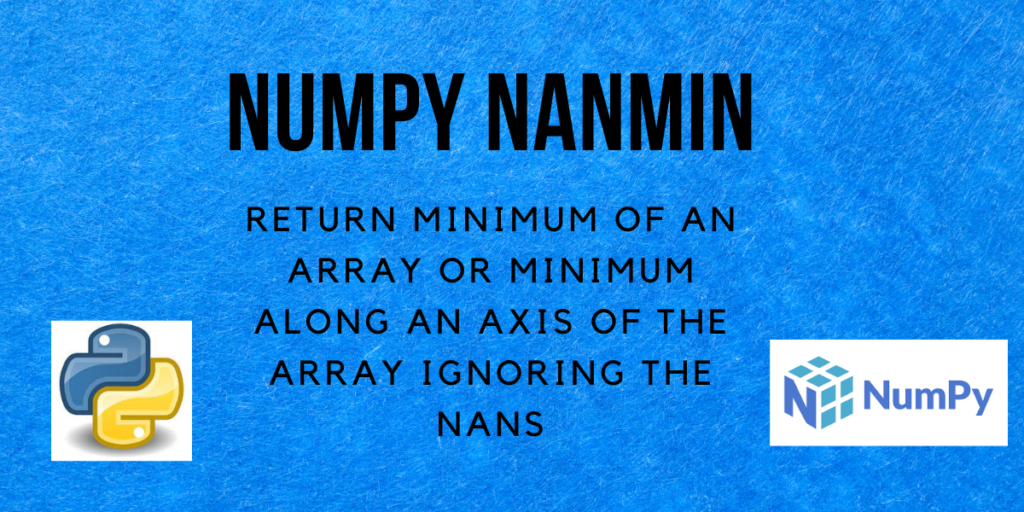# NumPy nanmin – Minimum of an array along an axis ignoring any NaNsHello and welcome to this tutorial on Numpy nanmin. In this tutorial, we will be learning about the NumPy `nanmin()` method and also seeing a lot of examples regarding the same. So let us begin!

Also read: NumPy nanmax – Maximum of an array along an axis ignoring any NaNs

## What is NumPy nanmin?

In Python, NaN denotes Not a Number. If we have an array that contains some NaN values and want to find the minimum value in it, we can use the `nanmin()` method from NumPy.

The `nanmin()` method in NumPy is a function that returns the minimum of the array elements calculated by ignoring the NaN values in the array. It can be the minimum of all the array elements, the minimum of the array elements along the rows or the minimum of the array elements along the columns.

We will see the examples for each of these in the upcoming section of this tutorial.

## Syntax of NumPy nanmin

Let us have a look at the syntax of the `nanmin()` function.

```numpy.nanmin(a, axis=None, out=None, keepdims=<no value>, initial=<no value>, where=<no value>)
```

Returns:
An array containing the minimum of the array along the specified axis, ignoring all the NaNs.

## Examples of NumPy nanmin

Let’s get right into the different examples of using numpy.nanmin() function.

### NumPy nanmin of a 1-d array

```import numpy as np

arr = [np.nan, 54, 1, 3, 44]
# using the nanmin function to calculate the maximum
ans = np.nanmin(arr)

print("arr =", arr)
print("Result =", ans)
```

Output:

```arr = [nan, 54, 1, 3, 44]
Result = 1.0
```

Ignoring the NaN value, the minimum value among 54, 1, 3 and 44 is 1, hence it is returned.

### NumPy nanmin of a 2-d array

```import numpy as np

arr = [[30, -9], [8, np.nan]]
# using the nanmin function to calculate the maximum
ans = np.nanmin(arr)

print("arr =", arr)
print("Result =", ans)
```

Output:

```arr = [[30, -9], [8, nan]]
Result = -9.0
```

Similar to the previous example, the minimum of 30, -9, and 8 is 8.

### NumPy nanmin along an axis of the array

axis = 0

```import numpy as np

arr = [[16, 4], [np.nan, 1]]
# using the nanmin function to calculate the maximum
ans = np.nanmin(arr, axis=0)

print("arr =", arr)
print("Result =", ans)
```

Output:

```arr = [[16, 4], [nan, 1]]
Result = [16.  1.]
```

Here, the values in each row for a specific column are compared to find the minimum element.

```ans = min(arr, arr) = min(16, np.nan) = 16 (ignoring NaN)
ans = min(arr, arr) = min(4, 1) = 1
```

axis = 1

```import numpy as np

arr = [[16, 4], [np.nan, 1]]
# using the nanmin function to calculate the maximum
ans = np.nanmin(arr, axis=1)

print("arr =", arr)
print("Result =", ans)
```

Output:

```arr = [[16, 4], [nan, 1]]
Result = [4. 1.]
```

When axis=1, the elements in each row are compared over all the columns to find the minimum.

```ans = min(arr, arr) = min(16, 4) = 4
ans = min(arr, arr) = min(np.nan, 1) = 1 (ignoring NaN)
```

### NumPy nanmin of an array containing infinity

Let us now see how the `numpy.nanmin()` method handles infinity along with NaNs in the array.

```import numpy as np

# array containing +infinity
a = np.array([16, 3, np.nan, 7, np.inf])
# array containing -infinity
b = np.array([16, 3, np.nan, 7, np.NINF])
# array containing +infinity and -infinity
c = np.array([16, 3, np.nan, np.NINF, 7, np.inf])

min_a = np.nanmin(a)
min_b = np.nanmin(b)
min_c = np.nanmin(c)

print("a =", a)
print("Minimum of the array a =", min_a)
print("\nb =", b)
print("Minimum of the array b =", min_b)
print("\nc =", c)
print("Minimum of the array c =", min_c)
```

Output:

```a = [16.  3. nan  7. inf]
Minimum of the array a = 3.0

b = [ 16.   3.  nan   7. -inf]
Minimum of the array b = -inf

c = [ 16.   3.  nan -inf   7.  inf]
Minimum of the array c = -inf
```

In the above code, NINF denotes -infinity and inf denotes infinity. Note that,

• If the array contains positive infinity then the minimum is the minimum of the whole array ignoring the NaNs.
• If the array contains negative infinity then the minimum is negative infinity.
• If the array contains both positive and negative infinity, then the minimum of the array is -inf, i.e. negative infinity.

## Conclusion

That’s all! In this tutorial, we learned about the Numpy nanmin method and practiced different types of examples using the same.